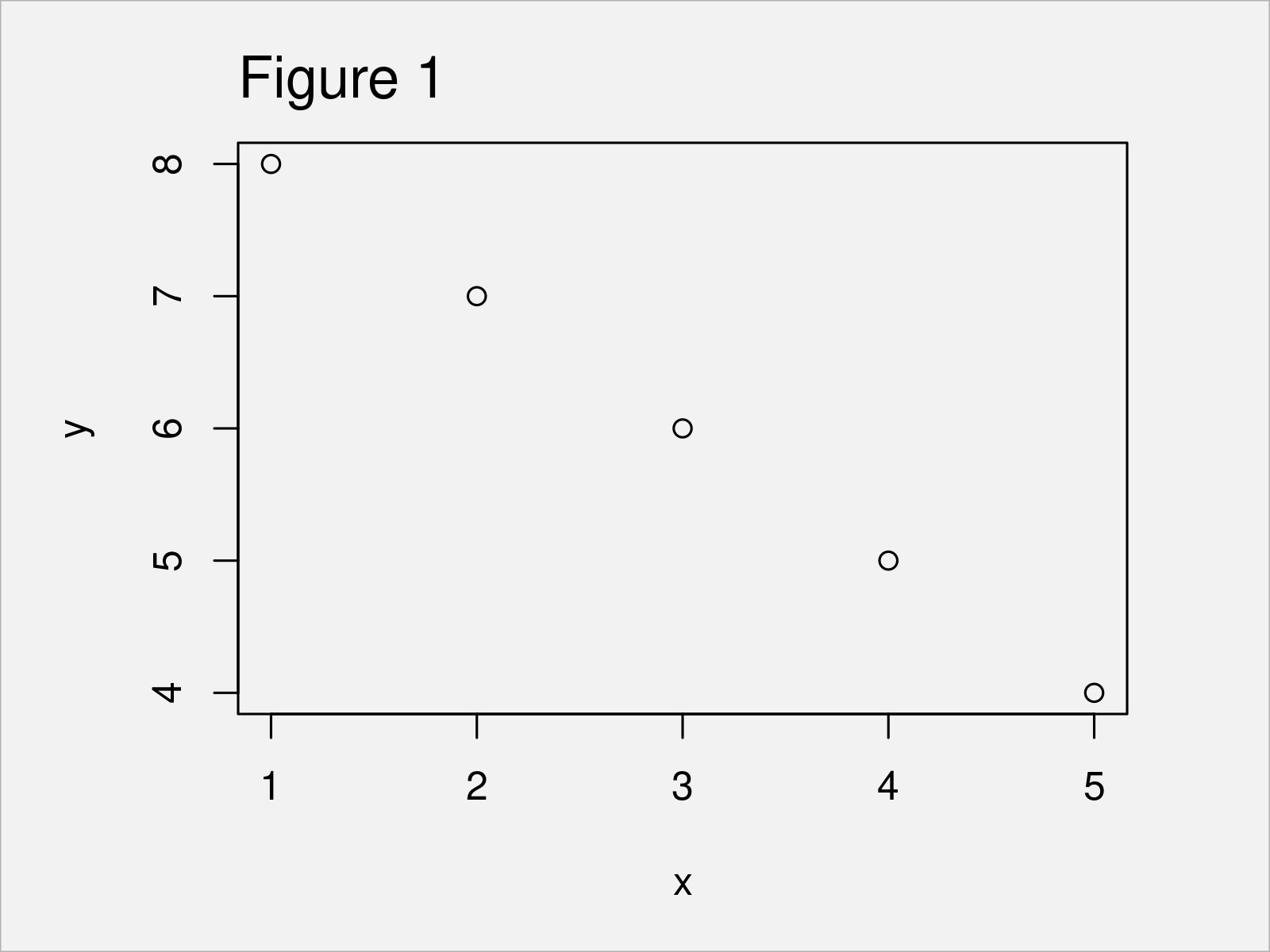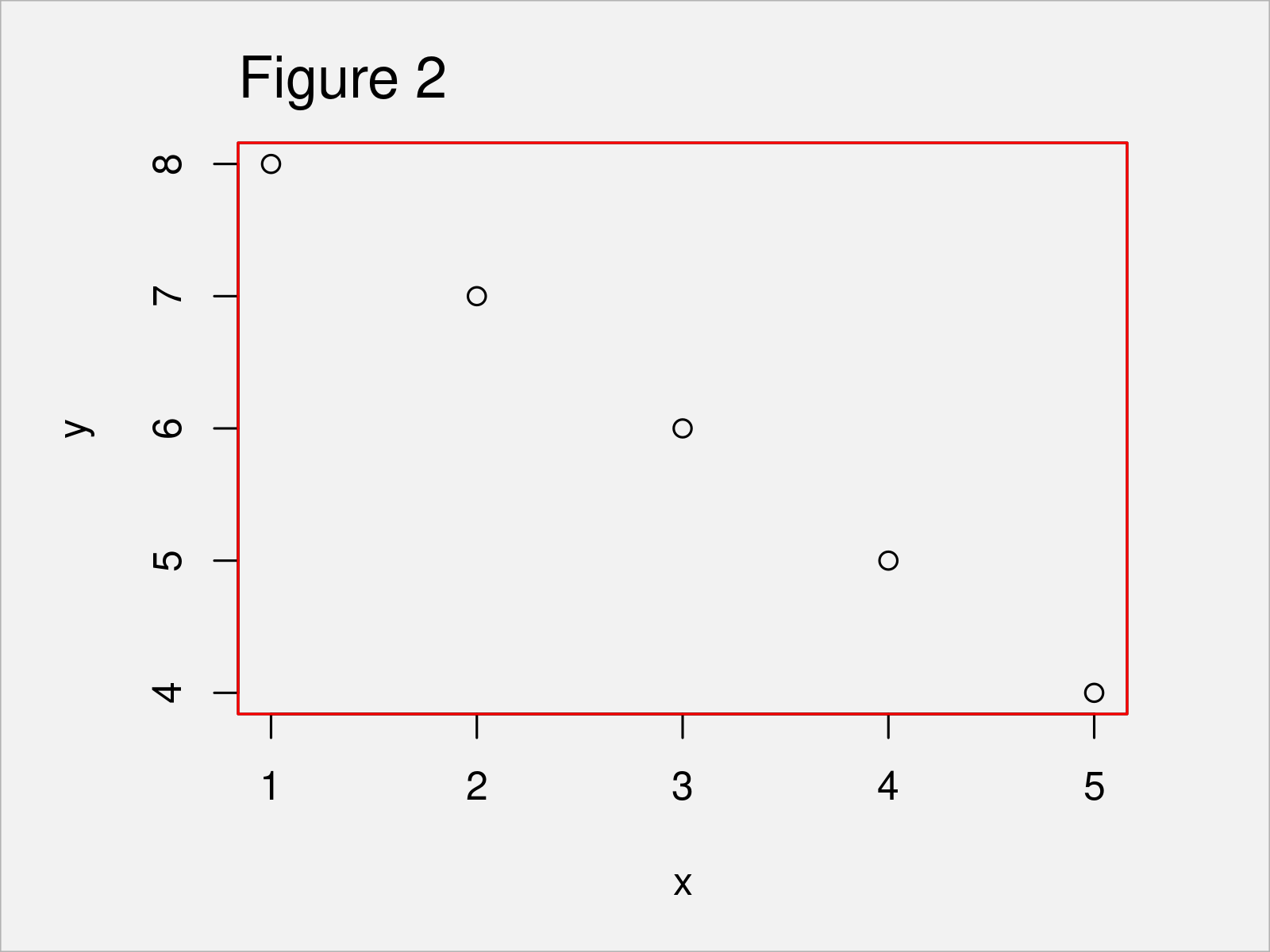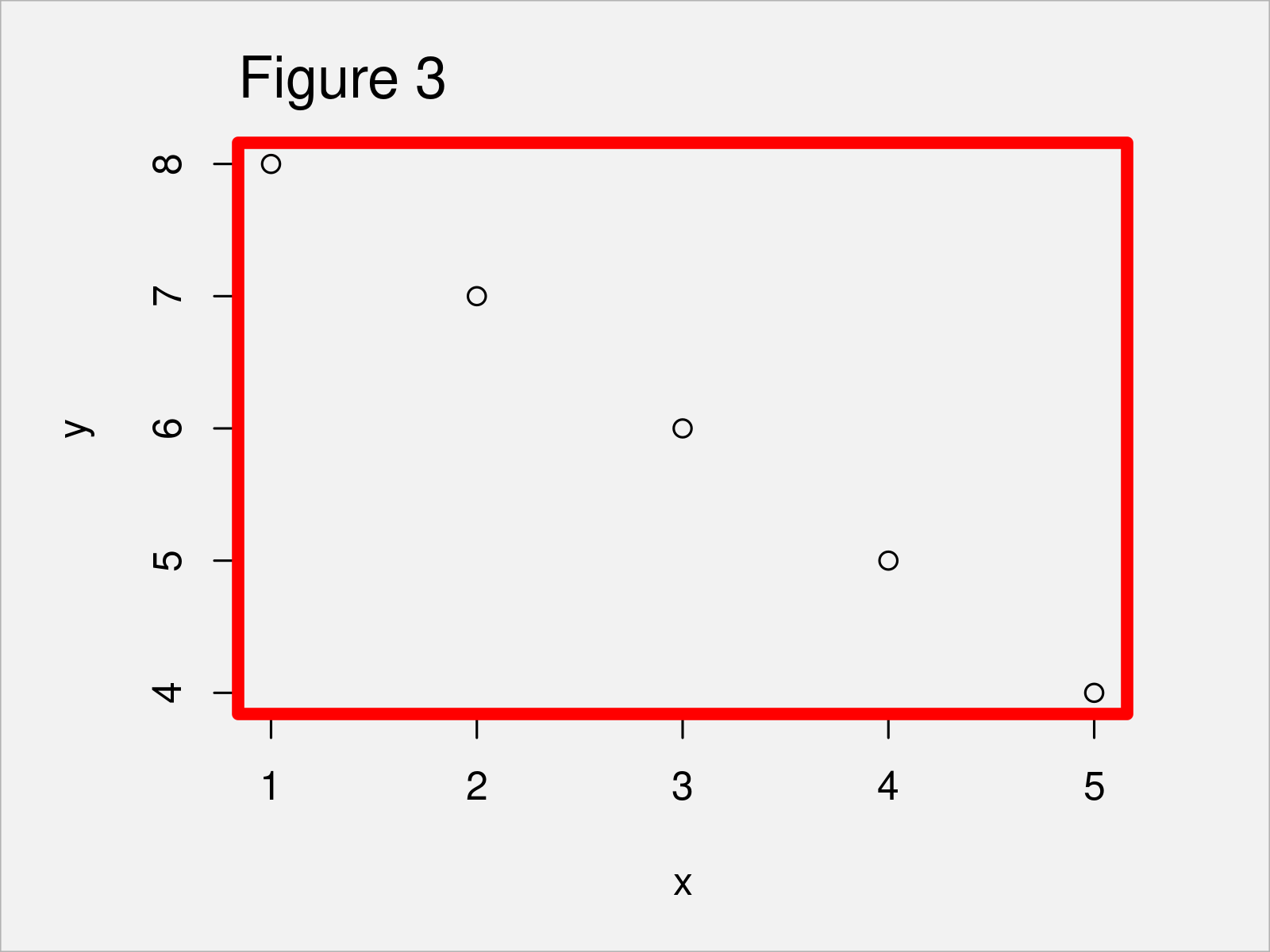# Draw Border Around Plot in Base R (3 Examples) | How to Apply the box() Function

In this R post you’ll learn how to draw a border around a plot using the box function.

The article looks as follows:

Here’s how to do it.

## Creation of Example Data

As the first step, I’ll need to create some example data:

```x <- 5:1                             # Create first example vector
x                                    # Print first example vector
#  5 4 3 2 1```
```y <- 4:8                             # Create second example vector
y                                    # Print second example vector
#  4 5 6 7 8```

As you can see based on the previously shown outputs of the RStudio console, we have created two example vectors containing five integer values each.

Now, we can create a graphic of the data as shown below:

`plot(x, y)                           # Draw plot`As illustrated in Figure 1, the previous R syntax has created a Base R scatterplot.

## Example 1: Add Box Around Plot

The following R syntax demonstrates how to add a colored box around the graphical panel of a plot.

For this task, we can apply the box function and the col argument as shown below:

```plot(x, y)                           # Draw plot
box(col = "red")                     # Add box to plot```In Figure 2 you can see that we have added a red border around the panel of our graph.

## Example 2: Change Thickness of Box Around Plot

The following code explains how to modify the thickness of the box around a Base R plot.

To do this, we have to increase or decrease the value specified to the lwd argument. The larger this value is, the thicker is the box around our plot:

```plot(x, y)                           # Draw plot
box(col = "red",                     # Add box to plot
lwd = 5)```After running the previous R syntax the box shown in Figure 3 has been created.

## Example 3: Add Box Around Entire Figure

In this example, I’ll illustrate how to draw a border around an entire graphic.

To achieve this, we have to specify the which argument to be equal to “figure”:

```plot(x, y)                           # Draw plot
box(col = "red",                     # Add box to plot
which = "figure")```Figure 4 shows the output of the previous R programming syntax: A scatterplot with box around the entire graph.

## Video, Further Resources & Summary

I have recently released a video tutorial on my YouTube channel, which illustrates the R syntax of this tutorial. Please find the video below.

Additionally, you could have a look at the related posts which I have published on this homepage:

To summarize: You have learned in this article how to add a border around a plot using the box function in the R programming language. Let me know in the comments, if you have additional questions.

Subscribe to the Statistics Globe Newsletter